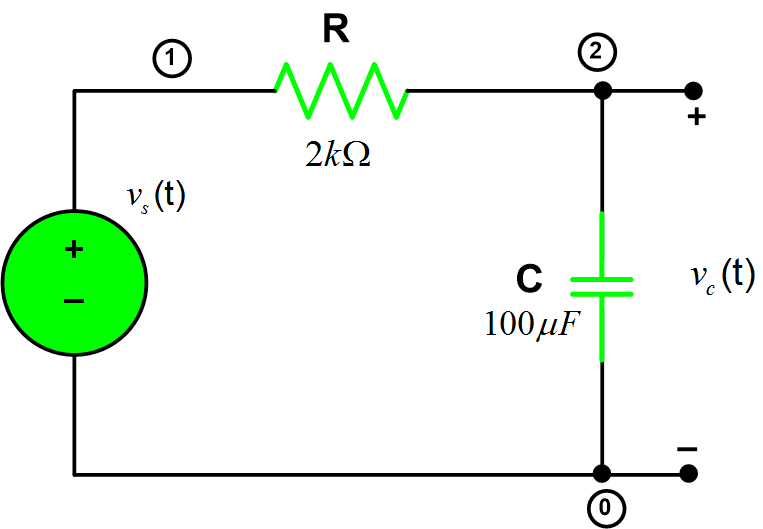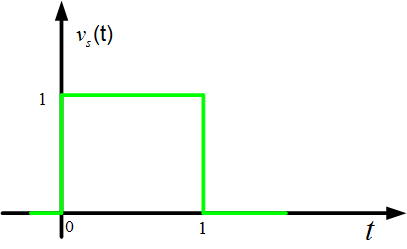# Current Through a Capacitor | Matlab

Want create site? Find Free WordPress Themes and plugins.

We can define the relationship between capacitor’s voltage and current as the amount of current flows through a capacitor depends on two factors: the capacitance and how rapidly the voltage is either ascending or descending. If the voltage goes up quickly, a large amount of current rushes through the capacitor. A slower rise up in voltage implies a little amount of current flows through it. If the voltage is constant, no current flows through it.

Determine the output voltage vc(t). Sketch vc(t).Let’s say circuit impulse response is:

$h(t)=\frac{1}{\tau }{{e}^{-t/\tau }}u(t)$

Input voltage as shown in figure, can be represented by the following expression:

${{v}_{s}}(t)=rect(t-0.5)V$

And output voltage can be written as:

${{v}_{c}}(t)=h(t)*{{v}_{s}}(t)$

Now, let’s try to write some code to compute the output voltage:

## Matlab Code for RC Circuit Charging and Discharging Analysis


%Convolution
clear all;close all;clc
%% Input Signal Data
T=1; %period of a signal
dt=T/500; % Sampling of a Signal
time_in=0:dt:2*T; % Time Interval for Input Signal graph
Amp=1; %Amplitude of a Signal
vs_in= Amp.*rect(time_in-T/2,T); % Source or Input Voltage
% Circuit Diagram Data
R= 2e3; % Resistance (2kOhm)
tau=R*C; % RC Series Circuit time constant
h_t=1./tau.*exp(-time_in./tau).*unitstep(time_in); % Impulse response h(t)
%% Output Signal vc(t) Calculation
vc_out=conv(h_t,vs_in).*dt; % Output Voltage
time_out=0:dt:4*T; % Time Interval for Output Signal graph
subplot(211)
plot(time_in,vs_in) % Plot for input signal vs(t)
xlabel('time (s)');ylabel('Amplitude (V)');title('v_s(t)');
axis([-0.2 2 -0.2 1.2]) % x and y axis limits
subplot(212)
plot(time_out,vc_out) % Plot for output signal vc(t)
xlabel('time (s)');ylabel('Amplitude (V)');title('v_c(t)');
axis([-0.2 2 -0.2 1.2]) % x and y axis limits
%==============================================


Function rect.m


function y=rect(t,tau)
%
% Rectangular function; y=rect(t,tau)
y=zeros(size(t));
I1=find(t>-tau/2 & t<=tau/2);
if (isempty(I1)~=1)
y(I1)=ones(size(I1));
end


Function unitstep.m


%=================== unitstep.m ===========
function y=unitstep(x)
%
% Unit-step function y=unitstep(x)
%
y=zeros(size(x));
I=find(x>0);
if (isempty(I)==0)
y(I)=ones(size(I));
end
%==============================================

Did you find apk for android? You can find new Free Android Games and apps.